# Solution assignment 09 Exponential functions and graphs

### Assignment 9

Draw the graphs of the functions: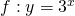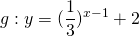Calculate the intersection point of both graphs.

### Solution

We already outlined in previous assignments how the graphs of both functions can be sketched.
To find the intersection point we have to solve the following equation: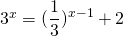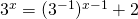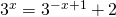We multiply both sides by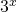and get: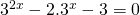We transform this equation by substituting: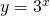Then we get: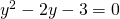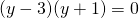We solved the quadratic equation by factorizing. The solutions of this equation are: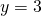or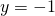. The latter soluton does not satisfy the equation because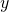is an exponential function and thus positive. Therefore we get the solution from the exponential equation: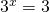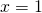From the figure we can verify that this solution is correct.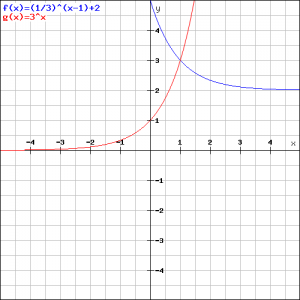0